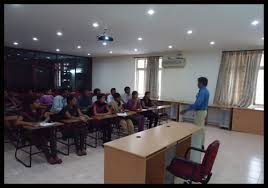## Timing Attacks on Implementations

Published on Jan 03, 2023

### Abstract

By carefully measuring the amount of time required to perform private key operations, attackers may be able to find fixed Diffie-Hellman exponents, factor RSA keys, and break other cryptosystems.Against a vulnerable system, the attack is computationally inexpensive and often requires only known ciphertext. Actual systems are potentially at risk, including cryptographic tokens, network-based cryptosystems, and other applications where attackers can make reasonably accurate timing measurements.

Techniques for preventing the attack for RSA and Diffie-Hellman are presented. Some cryptosystems will need to be re-vised to protect against the attack, and new protocols and algorithm may need to incorporate measures to prevent timing attacks. Keywords: timing attack, cryptanalysis, RSA, Diffie-Hellman, DSS.

### Introduction

Cryptosystems often take slightly different amounts of time to process different inputs. Reasons include performance optimizations to bypass unnecessary operations, branching and conditional statements, RAM cache hits, processor in-structions (such as multiplication and division) that run in non-fixed time, and a wide variety of other causes. Performance characteristics typically depend on both the encryption key and the input data (e.g., plaintext or ciphertext).

While it is known that timing channels can leak data or keys across a controlled perime-ter, intuition might suggest that unintentional timing characteristics would only reveal a small amount of information from a cryptosystem (such as the Hamming weight of the key). However, attacks are presented which can exploit timing measurements from vulnerable systems to find the entire secret key.

### Cryptanalysis of a Simple Modular Exponentiator

Diffe-Hellman and RSA private-key operations consist of computing R = yx mod n, where n is public and y can be found by an eavesdropper. The at-tacker's goal is to find x, the secret key. For the attack, the victim must com-pute yx mod n for several values of y, where y, n, and the computation time are known to the attacker. (If a new secret exponent x is chosen for each operation, the attack does not work.)

The necessary information and timing measurements might be obtained by passively eavesdropping on an interactive protocol, since an attacker could record the messages received by the target and measure the amount of time taken to respond to each y. The attack assumes that the attacker knows the design of the target system, although in practice this could probably be inferred from timing information.

### Montgomery Multiplication and the CRT

Modular reduction steps usually cause most of the timing variation in a modu-lar multiplication operation. Montgomery multiplication eliminates the mod n reduction steps and, as a result, tends to reduce the size of the timing character-istics. However, some variation usually remains. If the remaining \signal" is not dwarfed by measurement errors, the variance in tb and the variance of Pw_1 i=b+1 ti would be reduced proportionally and the attack would still work. However if the measurement error e is large, the required number of samples will increase in proportion to 1 Var(ti).

The Chinese Remainder Theorem (CRT) is also often used to optimize RSA private key operations. With CRT, (y mod p) and (y mod q) are computed first, where y is the message. These initial modular reduction steps can be vulnerable to timing attacks. The simplest such attack is to choose values of y that are close to p or q, then use timing measurements to determine whether the guessed value is larger or smaller than the actual value of p (or q). If y is less than p, computing y mod p has no e_ect, while if y is larger than p, it is necessary to subtract p from y at least once. Also, if the message is very slightly larger than p, y mod p will have leading zero digits, which may reduce the amount of time required for the first multiplication step.

 Are you interested in this topic.Then mail to us immediately to get the full report. email :- contactv2@gmail.com